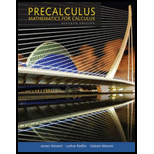Chapter 2.3, Problem 64E### Precalculus: Mathematics for Calcu...

7th Edition
James Stewart + 2 others
ISBN: 9781305071759

#### Solutions

Chapter
Section### Precalculus: Mathematics for Calcu...

7th Edition
James Stewart + 2 others
ISBN: 9781305071759
Textbook Problem

# Volume of Water Between 0°C and 30°C, the volume V (in cubic centimeters) of 1 kg of water at a temperature T is given by the formula V = 999 . 87 − 0.0 6426 T + 0.00 85 0 43 T 2 − 0.0000 679 T 3 Find the temperature at which the volume of 1 kg of water is a minimum.[Source: Physics, by D. Halliday and R. Resnick]

To determine

The temperature at which volume of 1 kg of water is minimum.

Explanation

The volume V of 1 kg of water at a temperature T between 0°C and 30°C is given by the formula,

V=999.870.06426T+0.0085043T20.0000679T3

Use the graph calculator to draw a graph of function V as shown below in Figure (1).

Figure (1)

Locate the local minimum point between T=0° and T=30°

### Still sussing out bartleby?

Check out a sample textbook solution.

See a sample solution

#### The Solution to Your Study Problems

Bartleby provides explanations to thousands of textbook problems written by our experts, many with advanced degrees!

Get Started

## Additional Math Solutions

#### Find more solutions based on key concepts